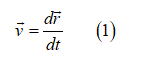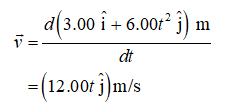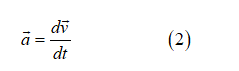# The vector position of a particle varies in time according to the expression r (3.00i 6.00t 2 j) m. (a) Findexpressions for the velocity and acceleration as functions of time. (b) Determine the particle’s position andvelocity at t 1.00 s

Question
6 views

The vector position of a particle varies in time according to the expression r (3.00i 6.00t 2 j) m. (a) Find
expressions for the velocity and acceleration as functions of time. (b) Determine the particle’s position and
velocity at t 1.00 s

check_circle

Formula for velocityDifferentiate the vector position w.r.t timeFormula for acceleration...

### Want to see the full answer?

See Solution

#### Want to see this answer and more?

Solutions are written by subject experts who are available 24/7. Questions are typically answered within 1 hour.*

See Solution
*Response times may vary by subject and question.
Tagged in